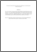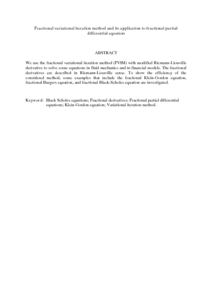Simple Search:

Fractional variational iteration method and its application to fractional partial differential equation

Citation

Elbeleze, Asma Ali and Kilicman, Adem and M. Taib, Bachok (2013) Fractional variational iteration method and its application to fractional partial differential equation. Mathematical Problems in Engineering, 2013. art. no. 543848. pp. 1-10. ISSN 1024-123X

Abstract / Synopsis

We use the fractional variational iteration method (FVIM) with modified Riemann-Liouville derivative to solve some equations in fluid mechanics and in financial models. The fractional derivatives are described in Riemann-Liouville sense. To show the efficiency of the considered method, some examples that include the fractional Klein-Gordon equation, fractional Burgers equation, and fractional Black-Scholes equation are investigated.Preview
PDF (Abstract)
Fractional variational iteration method and its application to fractional partial differential equation.pdfView Item